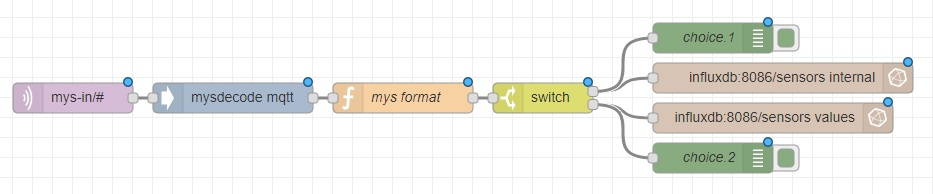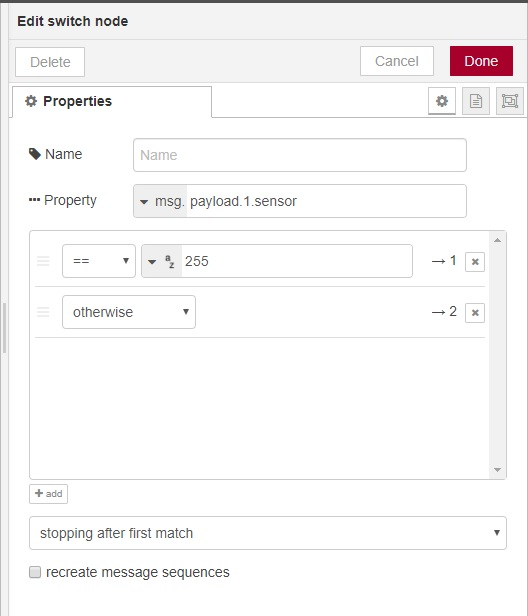# [Solved] problems trying to save on two measurements

• I'm trying to save the sensor data received from mqtt into two different measurements, if they are internal network messages, I want them in "internal" and all the sensor values into "values"
For that I tried using a switch module checking the "sensor" attribute being 255, but I can't make it save on the internal "table"To my confussion, the switch seems to work, as I put debug nodes on the output (1, 2) and it keeps printing 1 or 2 correctly depending on the message.
But from the two DB/measurement connections that hangs on it only executes the second one. Nothing is written into "internal", although the debug is hit and also the measurement has received the series:

``````key
---
internal,node=0,sensor=255,subType=I_HEARTBEAT_RESPONSE
internal,node=1,sensor=255,subType=I_DISCOVER_RESPONSE
values,node=1,sensor=1,subType=V_KWH
values,node=1,sensor=1,subType=V_VAR1
values,node=1,sensor=1,subType=V_WATT
values,node=1,sensor=2,subType=V_WATT
values,node=1,sensor=3,subType=V_WATT
values,node=1,sensor=4,subType=V_WATT
``````

Can someone give me a clue of what is happening? I'm newb with node-red and influxdb.

• Seems that the problem was that influxdb always configures the field type the first time a series is accessed.
I thought that the value was something like a "variant" and would eat anything I throw at it. So when I altered the data types in the flow, the db refused to save.
A drop and re create solved the problem.

3

1

2

36

6

5

9

2